• 岩石声学特性可以对岩石内部结构及力学特性进行描述与分类。通过对岩石声学特性研究可以得到岩石爆破破碎所需诸多信息。通过试验分析可知,声波速度与岩石爆破破碎效果密切相关,且岩石波形与岩石声波速度密切相关...
• 模型融合是比赛后期一个重要环节，大体来说有如下类型方式。 简单加权融合: 回归（分类概率）：算术平均融合（Arithmetic mean），几何平均融合（Geometric mean）； 分类：投票（Voting) ...5.3 相关
模型融合是比赛后期一个重要的环节，大体来说有如下的类型方式。

简单加权融合:

回归（分类概率）：算术平均融合（Arithmetic mean），几何平均融合（Geometric mean）；
分类：投票（Voting)
综合：排序融合(Rank averaging)，log融合

stacking/blending:

构建多层模型，并利用预测结果再拟合预测。

boosting/bagging（在xgboost，Adaboost,GBDT中已经用到）:

多树的提升方法

5.3 相关理论介绍
stacking具体原理详解

https://www.cnblogs.com/yumoye/p/11024137.html
https://zhuanlan.zhihu.com/p/26890738

# 5.4.1 回归\分类概率-融合：

#### (1) 简单加权平均，结果直接融合
import numpy as np
import pandas as pd
from sklearn import metrics

## 生成一些简单的样本数据，test_prei 代表第i个模型的预测值
test_pre1 = [1.2, 3.2, 2.1, 6.2]
test_pre2 = [0.9, 3.1, 2.0, 5.9]
test_pre3 = [1.1, 2.9, 2.2, 6.0]

# y_test_true 代表第模型的真实值
y_test_true = [1, 3, 2, 6]

## 定义结果的加权平均函数
def Weighted_method(test_pre1,test_pre2,test_pre3,w=[1/3,1/3,1/3]):
Weighted_result = w*pd.Series(test_pre1)+w*pd.Series(test_pre2)+w*pd.Series(test_pre3)
return Weighted_result

# 各模型的预测结果计算MAE
print('Pred1 MAE:',metrics.mean_absolute_error(y_test_true, test_pre1))
print('Pred2 MAE:',metrics.mean_absolute_error(y_test_true, test_pre2))
print('Pred3 MAE:',metrics.mean_absolute_error(y_test_true, test_pre3))

## 根据加权计算MAE
w = [0.3,0.4,0.3] # 定义比重权值
Weighted_pre = Weighted_method(test_pre1,test_pre2,test_pre3,w)
print('Weighted_pre MAE:',metrics.mean_absolute_error(y_test_true, Weighted_pre))


可以发现加权结果相对于之前的结果是有提升的，这种我们称其为简单的加权平均。
还有一些特殊的形式，比如mean平均，median平均
## 定义结果的加权平均函数
def Mean_method(test_pre1,test_pre2,test_pre3):
Mean_result = pd.concat([pd.Series(test_pre1),pd.Series(test_pre2),pd.Series(test_pre3)],axis=1).mean(axis=1)
return Mean_result

Mean_pre = Mean_method(test_pre1,test_pre2,test_pre3)
print('Mean_pre MAE:',metrics.mean_absolute_error(y_test_true, Mean_pre))

## 定义结果的加权平均函数
def Median_method(test_pre1,test_pre2,test_pre3):
Median_result = pd.concat([pd.Series(test_pre1),pd.Series(test_pre2),pd.Series(test_pre3)],axis=1).median(axis=1)
return Median_result

Median_pre = Median_method(test_pre1,test_pre2,test_pre3)
print('Median_pre MAE:',metrics.mean_absolute_error(y_test_true, Median_pre))

Mean_pre MAE: 0.06666666666666693
Median_pre MAE: 0.07500000000000007

#### (2) Stacking融合(回归)
from sklearn import linear_model

def Stacking_method(train_reg1,train_reg2,train_reg3,y_train_true,test_pre1,test_pre2,test_pre3,model_L2= linear_model.LinearRegression()):
model_L2.fit(pd.concat([pd.Series(train_reg1),pd.Series(train_reg2),pd.Series(train_reg3)],axis=1).values,y_train_true)
Stacking_result = model_L2.predict(pd.concat([pd.Series(test_pre1),pd.Series(test_pre2),pd.Series(test_pre3)],axis=1).values)
return Stacking_result

## 生成一些简单的样本数据，test_prei 代表第i个模型的预测值
train_reg1 = [3.2, 8.2, 9.1, 5.2]
train_reg2 = [2.9, 8.1, 9.0, 4.9]
train_reg3 = [3.1, 7.9, 9.2, 5.0]
# y_test_true 代表第模型的真实值
y_train_true = [3, 8, 9, 5]

test_pre1 = [1.2, 3.2, 2.1, 6.2]
test_pre2 = [0.9, 3.1, 2.0, 5.9]
test_pre3 = [1.1, 2.9, 2.2, 6.0]

# y_test_true 代表第模型的真实值
y_test_true = [1, 3, 2, 6]

model_L2= linear_model.LinearRegression()
Stacking_pre = Stacking_method(train_reg1,train_reg2,train_reg3,y_train_true,
test_pre1,test_pre2,test_pre3,model_L2)
print('Stacking_pre MAE:',metrics.mean_absolute_error(y_test_true, Stacking_pre))

Stacking_pre MAE: 0.04213483146067476

可以发现模型结果相对于之前有进一步的提升，这是我们需要注意的一点是，对于第二层Stacking的模型不宜选取的过于复杂，这样会导致模型在训练集上过拟合，从而使得在测试集上并不能达到很好的效果。
### 5.4.2 分类模型融合
import numpy as np
import lightgbm as lgb
from sklearn.datasets import make_blobs
from sklearn import datasets
from sklearn.tree import DecisionTreeClassifier
from sklearn.ensemble import RandomForestClassifier
from sklearn.ensemble import VotingClassifier
from sklearn.linear_model import LogisticRegression
from sklearn.svm import SVC
from sklearn.model_selection import train_test_split
from sklearn.datasets import make_moons
from sklearn.metrics import accuracy_score,roc_auc_score
from sklearn.model_selection import cross_val_score
from sklearn.model_selection import StratifiedKFold

#### (1) Voting投票机制

#Voting即投票机制，分为软投票和硬投票两种，其原理采用少数服从多数的思想。
'''
硬投票：对多个模型直接进行投票，不区分模型结果的相对重要度，最终投票数最多的类为最终被预测的类。
'''
iris = datasets.load_iris()

x=iris.data
y=iris.target
x_train,x_test,y_train,y_test=train_test_split(x,y,test_size=0.3)

clf1 = lgb.LGBMClassifier(learning_rate=0.1, n_estimators=150, max_depth=3, min_child_weight=2, subsample=0.7,
colsample_bytree=0.6, objective='binary:logistic')
clf2 = RandomForestClassifier(n_estimators=200, max_depth=10, min_samples_split=10,
min_samples_leaf=63,oob_score=True)
clf3 = SVC(C=0.1)

# 硬投票
eclf = VotingClassifier(estimators=[('lgb', clf1), ('rf', clf2), ('svc', clf3)], voting='hard')
for clf, label in zip([clf1, clf2, clf3, eclf], ['LGB', 'Random Forest', 'SVM', 'Ensemble']):
scores = cross_val_score(clf, x, y, cv=5, scoring='accuracy')
print("Accuracy: %0.2f (+/- %0.2f) [%s]" % (scores.mean(), scores.std(), label))

Accuracy: 0.95 (+/- 0.05) [LGB]
Accuracy: 0.33 (+/- 0.00) [Random Forest]
Accuracy: 0.92 (+/- 0.03) [SVM]
Accuracy: 0.93 (+/- 0.02) [Ensemble]

(2) 分类的Stacking\Blending融合：
stacking是一种分层模型集成框架。
以两层为例，第一层由多个基学习器组成，其输入为原始训练集，第二层的模型则是以第一层基学习器的输出作为训练集进行再训练，从而得到完整的stacking模型, stacking两层模型都使用了全部的训练数据。
'''
5-Fold Stacking
'''
from sklearn.ensemble import RandomForestClassifier
from sklearn.ensemble import ExtraTreesClassifier,GradientBoostingClassifier
import pandas as pd
#创建训练的数据集
data_0 = iris.data
data = data_0[:100,:]

target_0 = iris.target
target = target_0[:100]

#模型融合中使用到的各个单模型
clfs = [LogisticRegression(solver='lbfgs'),
RandomForestClassifier(n_estimators=5, n_jobs=-1, criterion='gini'),
ExtraTreesClassifier(n_estimators=5, n_jobs=-1, criterion='gini'),
ExtraTreesClassifier(n_estimators=5, n_jobs=-1, criterion='entropy'),
GradientBoostingClassifier(learning_rate=0.05, subsample=0.5, max_depth=6, n_estimators=5)]

#切分一部分数据作为测试集
X, X_predict, y, y_predict = train_test_split(data, target, test_size=0.3, random_state=2020)

dataset_blend_train = np.zeros((X.shape, len(clfs)))
dataset_blend_test = np.zeros((X_predict.shape, len(clfs)))

#5折stacking
n_splits = 5
skf = StratifiedKFold(n_splits)
skf = skf.split(X, y)

for j, clf in enumerate(clfs):
#依次训练各个单模型
dataset_blend_test_j = np.zeros((X_predict.shape, 5))
for i, (train, test) in enumerate(skf):
#5-Fold交叉训练，使用第i个部分作为预测，剩余的部分来训练模型，获得其预测的输出作为第i部分的新特征。
X_train, y_train, X_test, y_test = X[train], y[train], X[test], y[test]
clf.fit(X_train, y_train)
y_submission = clf.predict_proba(X_test)[:, 1]
dataset_blend_train[test, j] = y_submission
dataset_blend_test_j[:, i] = clf.predict_proba(X_predict)[:, 1]
#对于测试集，直接用这k个模型的预测值均值作为新的特征。
dataset_blend_test[:, j] = dataset_blend_test_j.mean(1)
print("val auc Score: %f" % roc_auc_score(y_predict, dataset_blend_test[:, j]))

clf = LogisticRegression(solver='lbfgs')
clf.fit(dataset_blend_train, y)
y_submission = clf.predict_proba(dataset_blend_test)[:, 1]

print("Val auc Score of Stacking: %f" % (roc_auc_score(y_predict, y_submission)))

val auc Score: 1.000000
val auc Score: 0.500000
val auc Score: 0.500000
val auc Score: 0.500000
val auc Score: 0.500000
Val auc Score of Stacking: 1.000000

Blending，其实和Stacking是一种类似的多层模型融合的形式

其主要思路是把原始的训练集先分成两部分，比如70%的数据作为新的训练集，剩下30%的数据作为测试集。
在第一层，我们在这70%的数据上训练多个模型，然后去预测那30%数据的label，同时也预测test集的label。
在第二层，我们就直接用这30%数据在第一层预测的结果做为新特征继续训练，然后用test集第一层预测的label做特征，用第二层训练的模型做进一步预测

其优点在于

比stacking简单（因为不用进行k次的交叉验证来获得stacker feature）
避开了一个信息泄露问题：generlizers和stacker使用了不一样的数据集

缺点在于：

使用了很少的数据（第二阶段的blender只使用training set10%的量）
blender可能会过拟合
stacking使用多次的交叉验证会比较稳健

'''
Blending
'''

#创建训练的数据集
#创建训练的数据集
data_0 = iris.data
data = data_0[:100,:]

target_0 = iris.target
target = target_0[:100]

#模型融合中使用到的各个单模型
clfs = [LogisticRegression(solver='lbfgs'),
RandomForestClassifier(n_estimators=5, n_jobs=-1, criterion='gini'),
RandomForestClassifier(n_estimators=5, n_jobs=-1, criterion='entropy'),
ExtraTreesClassifier(n_estimators=5, n_jobs=-1, criterion='gini'),
#ExtraTreesClassifier(n_estimators=5, n_jobs=-1, criterion='entropy'),
GradientBoostingClassifier(learning_rate=0.05, subsample=0.5, max_depth=6, n_estimators=5)]

#切分一部分数据作为测试集
X, X_predict, y, y_predict = train_test_split(data, target, test_size=0.3, random_state=2020)

#切分训练数据集为d1,d2两部分
X_d1, X_d2, y_d1, y_d2 = train_test_split(X, y, test_size=0.5, random_state=2020)
dataset_d1 = np.zeros((X_d2.shape, len(clfs)))
dataset_d2 = np.zeros((X_predict.shape, len(clfs)))

for j, clf in enumerate(clfs):
#依次训练各个单模型
clf.fit(X_d1, y_d1)
y_submission = clf.predict_proba(X_d2)[:, 1]
dataset_d1[:, j] = y_submission
#对于测试集，直接用这k个模型的预测值作为新的特征。
dataset_d2[:, j] = clf.predict_proba(X_predict)[:, 1]
print("val auc Score: %f" % roc_auc_score(y_predict, dataset_d2[:, j]))

#融合使用的模型
clf = GradientBoostingClassifier(learning_rate=0.02, subsample=0.5, max_depth=6, n_estimators=30)
clf.fit(dataset_d1, y_d2)
y_submission = clf.predict_proba(dataset_d2)[:, 1]
print("Val auc Score of Blending: %f" % (roc_auc_score(y_predict, y_submission)))

val auc Score: 1.000000
val auc Score: 1.000000
val auc Score: 1.000000
val auc Score: 1.000000
val auc Score: 1.000000
Val auc Score of Blending: 1.000000

### 5.4.3 一些其它方法

#将特征放进模型中预测，并将预测结果变换并作为新的特征加入原有特征中再经过模型预测结果 （Stacking变化）
#（可以反复预测多次将结果加入最后的特征中）
def Ensemble_add_feature(train,test,target,clfs):

# n_flods = 5
# skf = list(StratifiedKFold(y, n_folds=n_flods))

train_ = np.zeros((train.shape,len(clfs*2)))
test_ = np.zeros((test.shape,len(clfs*2)))

for j,clf in enumerate(clfs):
'''依次训练各个单模型'''
# print(j, clf)
'''使用第1个部分作为预测，第2部分来训练模型，获得其预测的输出作为第2部分的新特征。'''
# X_train, y_train, X_test, y_test = X[train], y[train], X[test], y[test]

clf.fit(train,target)
y_train = clf.predict(train)
y_test = clf.predict(test)

## 新特征生成
train_[:,j*2] = y_train**2
test_[:,j*2] = y_test**2
train_[:, j+1] = np.exp(y_train)
test_[:, j+1] = np.exp(y_test)
# print("val auc Score: %f" % r2_score(y_predict, dataset_d2[:, j]))
print('Method ',j)

train_ = pd.DataFrame(train_)
test_ = pd.DataFrame(test_)
return train_,test_

from sklearn.model_selection import cross_val_score, train_test_split
from sklearn.linear_model import LogisticRegression
clf = LogisticRegression()

data_0 = iris.data
data = data_0[:100,:]

target_0 = iris.target
target = target_0[:100]

x_train,x_test,y_train,y_test=train_test_split(data,target,test_size=0.3)
x_train = pd.DataFrame(x_train) ; x_test = pd.DataFrame(x_test)

#模型融合中使用到的各个单模型
clfs = [LogisticRegression(),
RandomForestClassifier(n_estimators=5, n_jobs=-1, criterion='gini'),
ExtraTreesClassifier(n_estimators=5, n_jobs=-1, criterion='gini'),
ExtraTreesClassifier(n_estimators=5, n_jobs=-1, criterion='entropy'),
GradientBoostingClassifier(learning_rate=0.05, subsample=0.5, max_depth=6, n_estimators=5)]

New_train,New_test = Ensemble_add_feature(x_train,x_test,y_train,clfs)

clf = LogisticRegression()
# clf = GradientBoostingClassifier(learning_rate=0.02, subsample=0.5, max_depth=6, n_estimators=30)
clf.fit(New_train, y_train)
y_emb = clf.predict_proba(New_test)[:, 1]

print("Val auc Score of stacking: %f" % (roc_auc_score(y_test, y_emb)))

Method  0
Method  1
Method  2
Method  3
Method  4
Val auc Score of stacking: 1.000000

5.5 本赛题示例
5.5.1 准备工作
准备工作进行内容有：

导入数据集并进行简单的预处理
将数据集划分成训练集和验证集
构建单模：Random Forest，LGB，NN
读取并演示如何利用融合模型生成可提交预测数据

import pandas as pd
import numpy as np
import warnings
import matplotlib
import matplotlib.pyplot as plt
import seaborn as sns

warnings.filterwarnings('ignore')
%matplotlib inline

import itertools
import matplotlib.gridspec as gridspec
from sklearn import datasets
from sklearn.linear_model import LogisticRegression
from sklearn.neighbors import KNeighborsClassifier
from sklearn.naive_bayes import GaussianNB
from sklearn.ensemble import RandomForestClassifier,RandomForestRegressor
# from mlxtend.classifier import StackingClassifier
from sklearn.model_selection import cross_val_score, train_test_split
# from mlxtend.plotting import plot_learning_curves
# from mlxtend.plotting import plot_decision_regions

from sklearn.model_selection import StratifiedKFold
from sklearn.model_selection import train_test_split
from sklearn.model_selection import StratifiedKFold
from sklearn.model_selection import train_test_split
import lightgbm as lgb
from sklearn.neural_network import MLPClassifier,MLPRegressor
from sklearn.metrics import mean_squared_error, mean_absolute_error

#这里引入一个降内存的函数。

def reduce_mem_usage(df):
start_mem = df.memory_usage().sum() / 1024**2
print('Memory usage of dataframe is {:.2f} MB'.format(start_mem))

for col in df.columns:
col_type = df[col].dtype

if col_type != object:
c_min = df[col].min()
c_max = df[col].max()
if str(col_type)[:3] == 'int':
if c_min > np.iinfo(np.int8).min and c_max < np.iinfo(np.int8).max:
df[col] = df[col].astype(np.int8)
elif c_min > np.iinfo(np.int16).min and c_max < np.iinfo(np.int16).max:
df[col] = df[col].astype(np.int16)
elif c_min > np.iinfo(np.int32).min and c_max < np.iinfo(np.int32).max:
df[col] = df[col].astype(np.int32)
elif c_min > np.iinfo(np.int64).min and c_max < np.iinfo(np.int64).max:
df[col] = df[col].astype(np.int64)
else:
if c_min > np.finfo(np.float16).min and c_max < np.finfo(np.float16).max:
df[col] = df[col].astype(np.float16)
elif c_min > np.finfo(np.float32).min and c_max < np.finfo(np.float32).max:
df[col] = df[col].astype(np.float32)
else:
df[col] = df[col].astype(np.float64)
else:
df[col] = df[col].astype('category')

end_mem = df.memory_usage().sum() / 1024**2
print('Memory usage after optimization is: {:.2f} MB'.format(end_mem))
print('Decreased by {:.1f}%'.format(100 * (start_mem - end_mem) / start_mem))

return df

train = pd.read_csv('./train.csv')
test = pd.read_csv('./testA.csv')

# 简单预处理
train_list = []
for items in train.values:
train_list.append([items] + [float(i) for i in items.split(',')] + [items])

test_list = []
for items in test.values:
test_list.append([items] + [float(i) for i in items.split(',')])

train = pd.DataFrame(np.array(train_list))
test = pd.DataFrame(np.array(test_list))

# id列不算入特征
features = ['s_'+str(i) for i in range(len(train_list)-2)]
train.columns = ['id'] + features + ['label']
test.columns = ['id'] + features

train = reduce_mem_usage(train)
test = reduce_mem_usage(test)

Memory usage of dataframe is 157.93 MB
Memory usage after optimization is: 39.67 MB
Decreased by 74.9%
Memory usage of dataframe is 31.43 MB
Memory usage after optimization is: 7.90 MB
Decreased by 74.9%

# 根据8：2划分训练集和校验集
X_train = train.drop(['id','label'], axis=1)
y_train = train['label']

# 测试集
X_test = test.drop(['id'], axis=1)

# 第一次运行可以先用一个subdata，这样速度会快些
X_train = X_train.iloc[:50000,:20]
y_train = y_train.iloc[:50000]
X_test = X_test.iloc[:,:20]

# 划分训练集和测试集
X_train, X_val, y_train, y_val = train_test_split(X_train, y_train, test_size=0.2)

# 单模函数
def build_model_rf(X_train,y_train):
model = RandomForestRegressor(n_estimators = 100)
model.fit(X_train, y_train)
return model

def build_model_lgb(X_train,y_train):
model = lgb.LGBMRegressor(num_leaves=63,learning_rate = 0.1,n_estimators = 100)
model.fit(X_train, y_train)
return model

def build_model_nn(X_train,y_train):
model = MLPRegressor(alpha=1e-05, hidden_layer_sizes=(5, 2), random_state=1,solver='lbfgs')
model.fit(X_train, y_train)
return model

# 这里针对三个单模进行训练，其中subA_rf/lgb/nn都是可以提交的模型
# 单模没有进行调参，因此是弱分类器，效果可能不是很好。

print('predict rf...')
model_rf = build_model_rf(X_train,y_train)
val_rf = model_rf.predict(X_val)
subA_rf = model_rf.predict(X_test)

print('predict lgb...')
model_lgb = build_model_lgb(X_train,y_train)
val_lgb = model_lgb.predict(X_val)
subA_lgb = model_rf.predict(X_test)

print('predict NN...')
model_nn = build_model_nn(X_train,y_train)
val_nn = model_nn.predict(X_val)
subA_nn = model_rf.predict(X_test)

predict rf...
predict lgb...
predict NN...

5.5.2 加权融合
首先我们尝试加权融合模型：

如果没有给权重矩阵，就是均值融合模型
权重矩阵可以进行自定义，这里我们是用三个单模进行融合。如果有更多需要更改矩阵size

# 加权融合模型，如果w没有变，就是均值融合
def Weighted_method(test_pre1,test_pre2,test_pre3,w=[1/3,1/3,1/3]):
Weighted_result = w*pd.Series(test_pre1)+w*pd.Series(test_pre2)+w*pd.Series(test_pre3)
return Weighted_result

# 初始权重，可以进行自定义，这里我们随便设置一个权重
w = [0.2, 0.3, 0.5]

val_pre = Weighted_method(val_rf,val_lgb,val_nn,w)
MAE_Weighted = mean_absolute_error(y_val,val_pre)
print('MAE of Weighted of val:',MAE_Weighted)

MAE of Weighted of val: 0.24455206510895008

#这里单独展示一下将多个单模预测结果融合成融和模型结果

## 预测数据部分
subA = Weighted_method(subA_rf,subA_lgb,subA_nn,w)

## 生成提交文件
sub = pd.DataFrame()
sub['SaleID'] = X_test.index
sub['price'] = subA
sub.to_csv('./sub_Weighted.csv',index=False)


### 5.5.3 Stacking融合
## Stacking

## 第一层
train_rf_pred = model_rf.predict(X_train)
train_lgb_pred = model_lgb.predict(X_train)
train_nn_pred = model_nn.predict(X_train)

stacking_X_train = pd.DataFrame()
stacking_X_train['Method_1'] = train_rf_pred
stacking_X_train['Method_2'] = train_lgb_pred
stacking_X_train['Method_3'] = train_nn_pred

stacking_X_val = pd.DataFrame()
stacking_X_val['Method_1'] = val_rf
stacking_X_val['Method_2'] = val_lgb
stacking_X_val['Method_3'] = val_nn

stacking_X_test = pd.DataFrame()
stacking_X_test['Method_1'] = subA_rf
stacking_X_test['Method_2'] = subA_lgb
stacking_X_test['Method_3'] = subA_nn

stacking_X_test.head()


Method_1
Method_2
Method_3

0
0.01
0.01
0.01

1
1.84
1.84
1.84

2
3.00
3.00
3.00

3
0.00
0.00
0.00

4
0.00
0.00
0.00

# 第二层是用random forest
model_lr_stacking = build_model_rf(stacking_X_train,y_train)

## 训练集
train_pre_Stacking = model_lr_stacking.predict(stacking_X_train)
print('MAE of stacking:',mean_absolute_error(y_train,train_pre_Stacking))

## 验证集
val_pre_Stacking = model_lr_stacking.predict(stacking_X_val)
print('MAE of stacking:',mean_absolute_error(y_val,val_pre_Stacking))

## 预测集
print('Predict stacking...')
subA_Stacking = model_lr_stacking.predict(stacking_X_test)

MAE of stacking: 0.0013057499999999996
MAE of stacking: 0.08504199999999999
Predict stacking...

5.6 经验总结
模型融合是数据挖掘比赛后期上分的主要方式，尤其是进行队伍合并后，模型融合有很多优势。总结一下三个方面：

结果层面的融合，这种是最常见的融合方法，其可行的融合方法也有很多，比如根据结果的得分进行加权融合，还可以做Log，exp处理等。在做结果融合的时候。有一个很重要的条件是模型结果的得分要比较近似但结果的差异要比较大，这样的结果融合往往有比较好的效果提升。如果不满足这个条件带来的效果很低，甚至是负效果。

特征层面的融合，这个层面叫融合融合并不准确，主要是队伍合并后大家可以相互学习特征工程。如果我们用同种模型训练，可以把特征进行切分给不同的模型，然后在后面进行模型或者结果融合有时也能产生比较好的效果。

模型层面的融合，模型层面的融合可能就涉及模型的堆叠和设计，比如加stacking，部分模型的结果作为特征输入等，这些就需要多实验和思考了，基于模型层面的融合最好不同模型类型要有一定的差异，用同种模型不同的参数的收益一般是比较小的。

以上内容来源于：Datawhale
Datawhale是一个专注于数据科学与AI领域的开源组织


展开全文python 机器学习
• 我们总会遇到图像处理中一些模糊概念，怎么也不清楚，说知道也知道，说不知道也不知道，就像隔了一纱，那今天就让我们一起来揭开她神秘面纱！！！ 一、Blob概念理解 1、概念理解 1 blob: n. ...
OpenCV中blob的概念以及OpenCV中BLOB特征提取与几何形状分类文章目录：一、Blob概念理解1、概念理解 12、概念理解2二、blob特征提取1、python2、C++

我们总会遇到图像处理中的一些模糊的概念，怎么也理不清楚，说知道也知道，说不知道也不知道，就像隔了一层纱，那今天就让我们一起来揭开她神秘的面纱！！！

一、Blob概念理解
1、概念理解 1
blob:  n.   /blɒb/    一点，一滴；（颜色的）一小片，斑点

可以直观的理解是色斑：就是相同像素组成的一小块，一小块的特征

Blob分析：（Blob Analysis）是对图像中相同像素的连通域进行分析，该连通域称为Blob。经二值化（Binary Thresholding）处理后的图像中色斑可认为是blob。Blob分析工具可以从背景中分离出目标，并可以计算出目标的数量、位置、形状、方向和大小，还可以提供相关斑点间的拓扑结构。在处理过程中不是对单个像素逐一分析，而是对图像的行进行操作。图像的每一行都用游程长度编码（RLE）来表示相邻的目标范围。这种算法与基于像素的算法相比，大大提高了处理的速度。
2、概念理解2
首先要了解，什么是blob特征，我们来看下面两幅图片。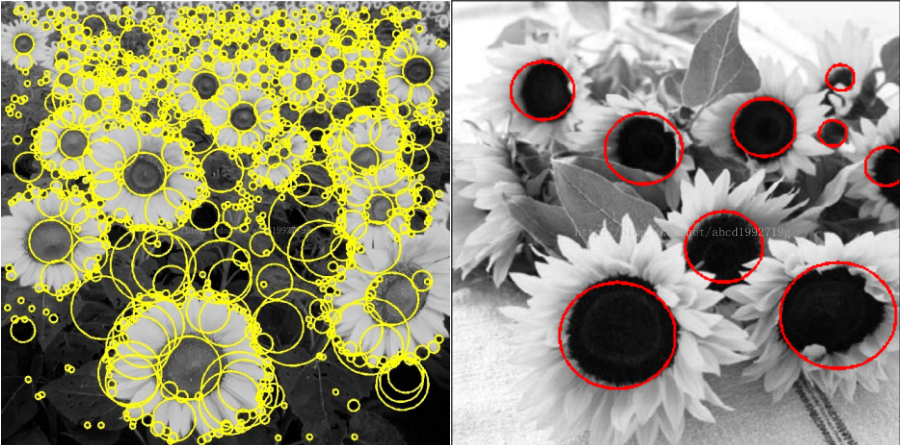直观上来看，blob特征就是一团，一坨东西，它并不一定是圆形的，总之它就是那么一团独立存在的特征。
二、blob特征提取
1、python
# Standard imports
import cv2
import numpy as np;
# Read image
im = cv2.imread("blob.jpg", cv2.IMREAD_GRAYSCALE)
# Set up the detector with default parameters.
detector = cv2.SimpleBlobDetector()
# Detect blobs.
keypoints = detector.detect(im)
# Draw detected blobs as red circles.
# cv2.DRAW_MATCHES_FLAGS_DRAW_RICH_KEYPOINTS ensures the size of the circle corresponds to the size of blob
im_with_keypoints = cv2.drawKeypoints(im, keypoints, np.array([]), (0,0,255), cv2.DRAW_MATCHES_FLAGS_DRAW_RICH_KEYPOINTS)
# Show keypoints
cv2.imshow("Keypoints", im_with_keypoints)
cv2.waitKey(0)

2、C++
using namespace cv;
// Read image
Mat im = imread( "blob.jpg", IMREAD_GRAYSCALE );
// Set up the detector with default parameters.
SimpleBlobDetector detector;
// Detect blobs.
std::vector<KeyPoint> keypoints;
detector.detect( im, keypoints);
// Draw detected blobs as red circles.
// DrawMatchesFlags::DRAW_RICH_KEYPOINTS flag ensures the size of the circle corresponds to the size of blob
Mat im_with_keypoints;
drawKeypoints( im, keypoints, im_with_keypoints, Scalar(0,0,255), DrawMatchesFlags::DRAW_RICH_KEYPOINTS );
// Show blobs
imshow("keypoints", im_with_keypoints );
waitKey(0);

Reference：
1、https://blog.csdn.net/abcd1992719g/article/details/27071273
2、https://blog.csdn.net/liyangblog/article/details/24962313
3、https://cloud.tencent.com/developer/article/1084326
4、https://brightguo.wordpress.com/2016/05/21/blob-detection-using-opencv-python-c/♠ ⊕ ♠ ⊕  ♠ ⊕ ♠ ⊕ ♠ ⊕  ♠ ⊕ ♠ ⊕ ♠ ⊕  ♠ ⊕ ♠ ⊕ ♠ ⊕  ♠ ⊕ ♠ ⊕ ♠ ⊕  ♠ ⊕ ♠ ⊕ ♠ ⊕  ♠ ⊕ ♠ ⊕ ♠ ⊕  ♠ ⊕ ♠ ⊕ ♠ ⊕  ♠ ⊕ ♠ ⊕ ♠ ⊕  ♠ ⊕ ♠ ⊕ ♠ ⊕  ♠ ⊕ ♠


展开全文opencv blob
•人工智能 机器学习
• 索引是什么? 索引是帮助MySQL高效获取数据的数据结构。 索引能干什么?...2. 从应用（使用过程中进行的分类）次来分： 普通索引（即该列（字段）的值 可以重复，MUL，multiply（多个））； ...
索引是什么?    索引能干什么?

索引是帮助MySQL高效获取数据的数据结构；提高数据查询的效率。

一、索引的分类

1. 从存储结构（索引存储时保存的形式）上来划分：

BTree索引（B-Tree或B+Tree索引），Hash索引，full-index全文索引，R-Tree索引。

2. 从应用层（使用过程中进行的分类）次来分：

普通索引（即该列（字段）的值 可以重复，MUL，multiply（多个））；

唯一索引（索引列的值必须唯一，不允许出现重复，但允许为空NULL，UNI，unique（独一无二））；

复合索引（即一个索引包含多个列）。

3. 从数据的物理顺序与键值的逻辑（索引）顺序关系：

聚集索引（定义：表的物理顺序与键值的索引顺序一致；由于聚集索引规定数据在表中的物理存储顺序，因此一个表只能包含一个聚集索引，但该索引可以包含多个列(组合索引。类似于复合索引)；并不是一种单独的索引类型，而是一种数据存储方式。具体细节取决于不同的实现，InnoDB的聚簇索引其实就是在同一个结构中保存了B-Tree索引(技术上来说是B+Tree)和数据行；（主键，即值不能重复，且不能为空的索引，是建立了唯一的聚集或者非聚集索引）。

非聚集索引

二、索引的底层实现

问：为什么索引结构默认使用B-Tree，而不是hash，二叉树，红黑树？

hash：虽然可以快速定位，但是没有顺序，IO复杂度高。二叉树：树的高度不均匀，不能自平衡，查找效率跟数据有关（树的高度），并且IO代价高。

红黑树：树的高度随着数据量增加而增加，IO代价高。

问：为什么官方建议使用自增长主键作为索引。

结合B+Tree的特点，自增主键是连续的，在插入过程中尽量减少页分裂，即使要进行页分裂，也只会分裂很少一部分。并且能减少数据的移动，每次插入都是插入到最后。

总之就是减少分裂和移动的频率。

插入连续的数据：                                                          插入非连续的数据：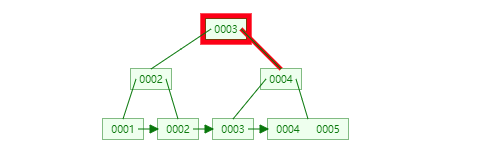展开全文•人工智能 机器学习
•人工智能 机器学习
• 清楚数据间关系，或者拟合数据分布。 两或者以上神经网络，几乎可以拟合任意函数 做训练，目的就在于：通过训练，我找到可以理清数据间关系神经网络，或者拟合数据分布神经网络 训练前，用模型提取...
1、训练（Training)
模型训练的目的：给数据作分类.
kernel:要么找到数据的因果关系，要么找到数据间的关联。理清楚数据间的关系，或者拟合数据的分布。
两层或者以上的神经网络，几乎可以拟合任意函数
做训练，目的就在于：通过训练，我找到可以理清数据间关系的神经网络，或者拟合数据分布的神经网络
训练前，用模型提取出来的特征分布图， 可以看到不同数据之间的特征‘纠缠’在一起，难分难解。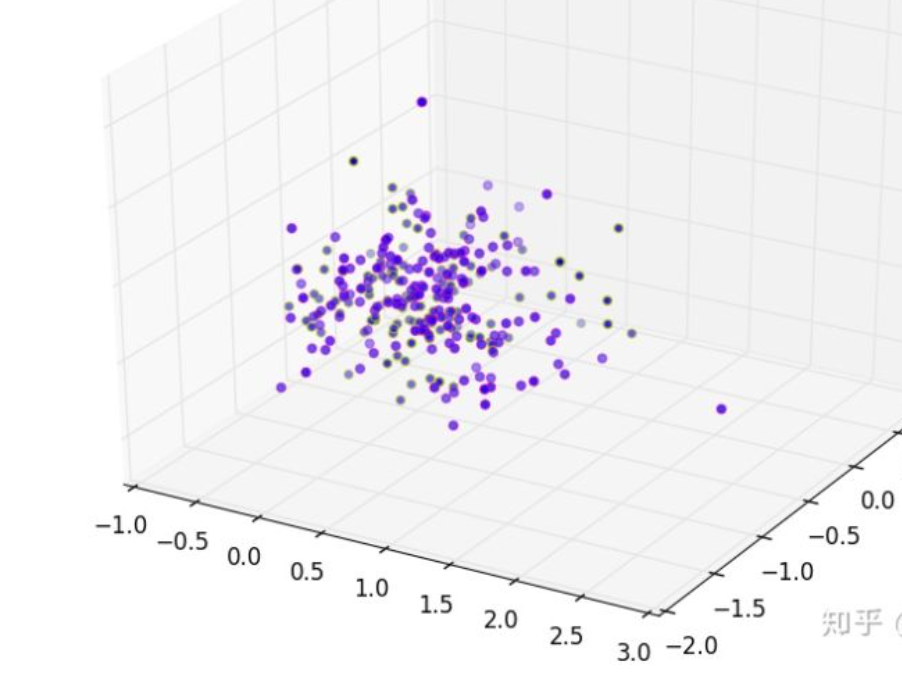经过足够的训练之后，你应该能明显看到特征甚至已肉眼可分的方式，被区分开来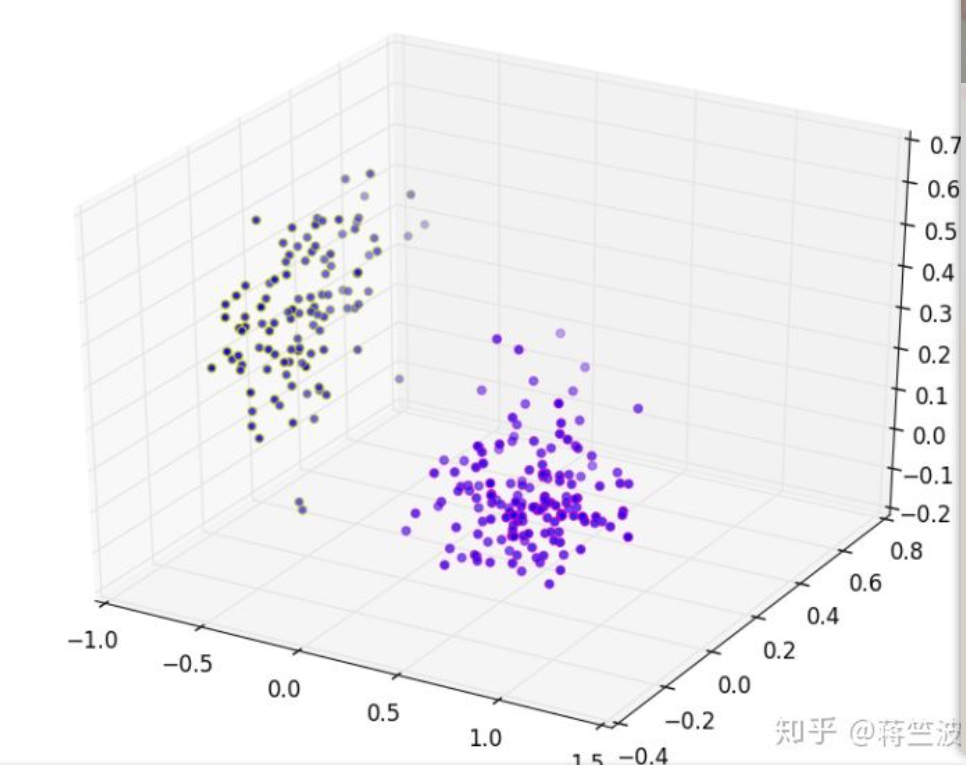2、采样层(Pooling)
卷积输出了，输出的特征图（feature map）到了采样（pooling，有些也叫subsample）层手上，采样层实际上就是一个特征选择的过程。
假设我们用边缘滤波器去卷积输入图片，得到的特征值矩阵如下：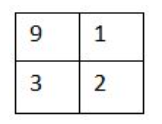采样层(pooling)非常好理解，我们这里特指maxpooling，Maxpooling 就是在这个区域内选出最能代表边缘的值，也就是9，然后丢掉那些没多大用的信息。
池化：非常类似卷积层的卷积核。可以理解为卷积核每空两格做一次卷积，卷积核的大小是2x2， 但是卷积核的作用是取这个核里面最大的值（即特征最明显的值），而不是做卷积运算。
可以一定程度提高空间不变性，比如说平移不变性，尺度不变性，形变不变性
Pooling 层说到底还是一个特征选择，信息过滤的过程，也就是说我们损失了一部分信息，这是一个和计算性能的一个妥协，随着运算速度的不断提高，我觉得这个妥协会越来越小。现在有些网络都开始少用或者不用pooling层了
特征提取的误差主要来自两个方面：
（1）邻域大小受限；
（2）卷积层权值参数误差。
average pooling 和max-pooling 的主要区别在于：
average -pooling能减小第一种误差，更多的保留图像的背景信息。
max-pooling能减小第二种误差，更多的保留纹理信息。
3、激活函数（Activation Function)
实际上，从下采样层出来的结果，不是又到卷积层，而是进入了一个激活函数（activation function）。
最简单的二分类非线性激活函数开始—阶跃函数（Step Function）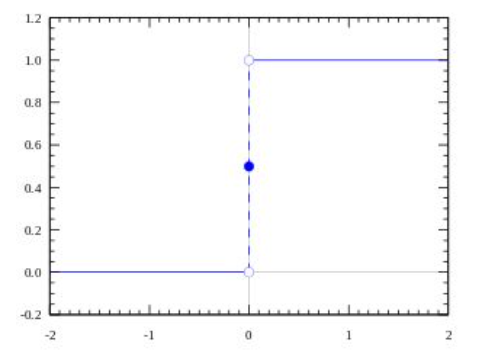当输入（也就是神经网络Y的输出）大于0就被分类到1（100% 被激活 ），小于0就分到0，（没有被激活）。
可是激活值只有100%或者0%则存在误差，我们希望它可以是0%—100%任意值值越大，激活程度越高 对于分类，也就意味着它属于这一类的概率越大。
经常听到或者用到的激活函数有：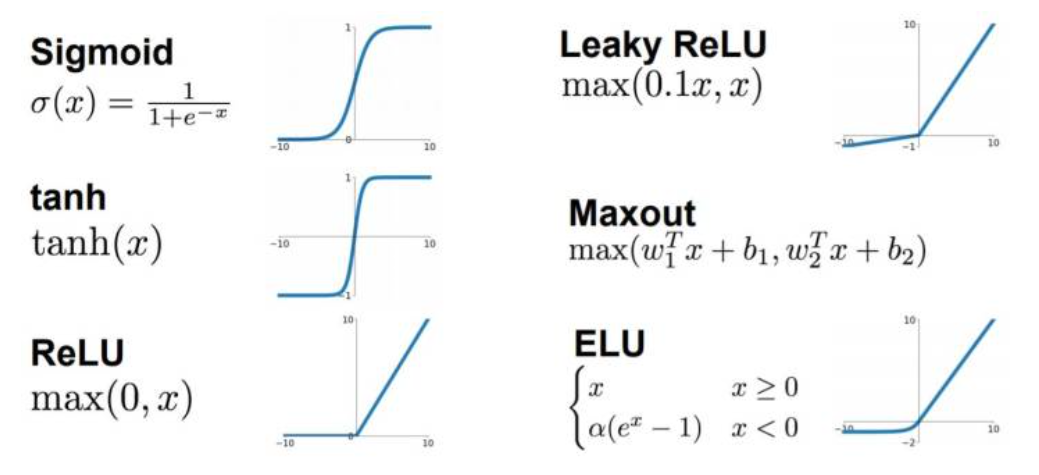sigmoid 只会输出正数，以及靠近0的输出变化率最大，tanh和sigmoid不同的是，tanh输出可以是负数，ReLu是输入只能大于0,如果你输入含有负数，ReLu就不适合，如果你的输入是图片格式，ReLu就挺常用的。
激活函数确实是很好宽广的点，它对于提高模型鲁棒性，非线性表达能力，缓解梯度消失问题，将特征图映射到新的特征空间从何更有利于训练，加速模型收敛等问题都有很好的帮助
4、Dropout
这种情况就是dropout rate 设置太大，我们这是0.5，在训练的时候不幸丢掉了重点特征，造成模型overfitting.一般情况，dropout rate 设为0.3-0.5即可
一个模型的作用效果，也转变成4个模型的效果和，更像一个多模型投票。虽然第四个模型overfitting了，但是前三个也模型参与投票判断，第四个模型overfitting就被弱化了所以，dropout的出现，使得模型稳定性和鲁棒性被大大提高了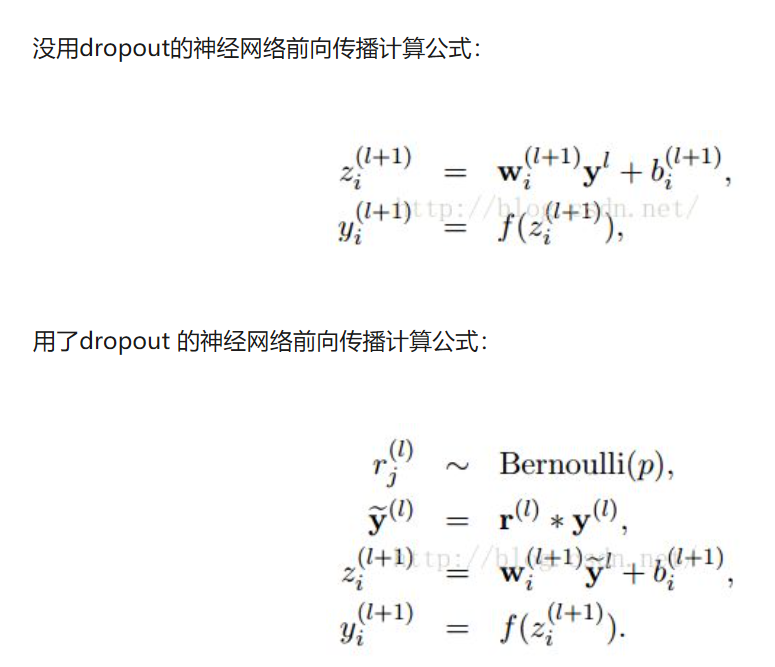`
展开全文计算机视觉
•人工智能 机器学习
• 理分类并对各类数据数据属性进行了规范。 2PDM体系结构、集成框架和主要功能标准中提出了适用于航空行业PDM体系结构和集成框架。其中将体系结构分为支撑、对象、功能和用户等四便于企业根据实际...
• 本文通过引入包括Isomap 流形降维、查询语义词典(WordNet) 等高度非线性方法,期望将文本信息处领域长期专注于“语法”层次研究,演进到“语义”层次. 利用流形学习工具研究了中文词汇在语义空间(分类空间) ...
• 其实，事情意义是可以被分类而因此出其中相互比较标准。如果我们能够把大部分时间和精力放在有深远意义事情上，累积出来效果，自然把人生推至更理想高线上。 这一章内容是NLP最能对人
• 在沉积期间和沉积之后到沉积物还未固结之前由上述作用所产生沉积构造被定义为“原生沉积构造”,包括各种类型的层理、层面上各种作用痕迹以及各种变形现象。在该分类之中,叠层石被定义为突出沉积面正生长构造,...
• 这是一张流传很广，并且很经典...通过这条线，可以出从发送端一直到接受端，报文是怎么一被封装。 根据不同层次中处理数据不同，可以对不同层次上物理设备进行分类，简单分类一下，对应下图。 ...
• ISO将整个网络通信的功能划分为7个层次(如图所示)常见的传输层协议在各个层理的分布如下了解 HTTP协议是基于Tcp/Ip协议,同时HTTP协议采用请求/响应模型浏览器或其他客户端发出请求，服务器给予响应(http协议永远都...http协议
• 最近在coursera上看...输入层的每个神经元代表一个特征，输出层的每个神经元代表一个分类标签，而隐层的层数和神经元数目则由人工设定。 一个典型3神经网络如图所示： 设第lll神经元个数为sls_lsl​，其中第i
• 及其与内核交互.最后给出了Netfilter 构建防火墙一个实例。 关键词：防火墙 Linux Netfilter 包过滤 中图分类号：TN393 文献标识码：A Study of the technology of firewall based on Linux SONG Wen-gong1 ...
• 2.4.2　配置的分类 33 2.4.3　应用程序的配置管理 33 2.4.4　跨应用的配置管理 36 2.4.5　管理配置信息的原则 37 2.5　环境管理 38 2.5.1　环境管理的工具 41 2.5.2　变更过程管理 41 2.6　小结 42 第3章　...
• ，比较各类算法执行时细微差别大有禅益。具备大学数学基础和高级程序设计语言知识人均可自学本书。 　本书适合专业程序员、工程师及科研人员使用。非常适用于作为为高年级本科生和一年级研究生开设重点讲授...
• 研究隧道掌子面地质信息数字编录识别技术是为了发展隧道掌子面地质编录法，它主要是利用数字图像处理技术代替地质素描图，利用图像处理模式识别技术代替专业人员对岩体节理、裂隙、层理等微构造发育情况分析认识...
• 决策树结构很像二叉树，通过一层的节点，来对我们样本进行分类。决策树算法可解释性非常好，通过绘制决策树，我们可以很清楚地解算法工作原理，同时也方便向别人进行展示。这一节，我们重点是画...决策树 机器学习 python
• 首先一下网络输出shape，和数据labelshape。再看一下计算loss时候应该怎么把数据转换成容易计算形式。 1 shape 1.1 网络输出shape 下图表示分类分支预测输出feature map。 格子表示featuremap上像素...retinanet
• linux面试题 文章目录linux面试题1. 网络服务相关面试题：1. 面试题：简述tcp三次握手的过程2.... 面试题：ip地址的分类私有ip地址分类：2.linux系统管理相关面试题1. 面试题：谈谈你对liunx权限的...linux 运维
• 开篇 同样作为深度学习入门系统博客吧，希望闲时能够花个不到一个小时一下吴恩达老师课程里面...常见激活函数，缺点就不提了，二分类任务输出比较常见，下面是它函数图 它实现 import math de...吴恩达
• 5层理：指沉积岩成分、颜色和结构沿垂直方向发生变化而表现出来层状构造。 6解理：矿物晶体在外力作用下，沿一定方向破裂成光滑平面性质，称为解理。 8地层：是地壳发展过程中所形成岩层总称。 9地层单位：...
•  转眼间，看usb gadget代码看有一段时间了，把其中层次关系大致的理了一遍，就写出来和大家分享下，不对之处敬请指出，了解层次结构嘛，当然是代码越简单越好，俗话说“柿子专拣软捏”，就拿最简单zero.c...
• 2012-12-11 21:30 5187人阅读 评论(1) 收藏 举报  分类： ...LINUX 内核中 SCSI 子系统由 SCSI 上层，中间和底层驱动模块  三部分组成，主要负责管理 SCSI ...因此，理解 SCSI 子系统 IO 处理机制对linux SCSI
• ## 计算机操作系统(第三版)

千次下载 热门讨论 2013-08-13 17:19:05
3.4.2　实时调度算法的分类 99 3.4.3　常用的几种实时调度算法 100 3.5　产生死锁的原因和必要条件 103 3.5.1　产生死锁的原因 103 3.5.2　产生死锁的必要条件 105 3.5.3　处理死锁的基本方法 105 3.6　预防...
• 选择分类后，按摇 按左向键收缩已展开的 分类,按摇按右向键展 开已收缩的分类. 3.项目明细信息查看途径 1).选择项目后按摇按 选择键使项目进入明细 信息显示状态，当再次 按下该键...
• 首先说说我们在应用中那些情况会用到Switch或者说用Switch比较好 场景： switch语句是多分支选择语句.用来实现多分支选择...当然这些都可以用嵌套if语句来外,但如果分支较多,则嵌套if语句 数多,程序沉...Switch用法 java基础